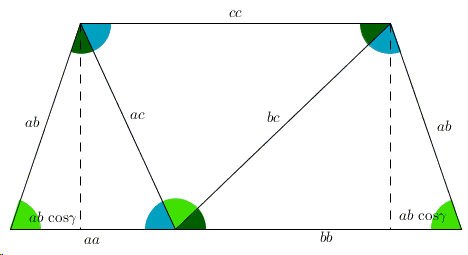# The Law of Cosines - Another PWW

The applet below illustrates a proof without words of the Law of Cosines that establishes a relationship between the angles and the side lengths of $\Delta ABC$:

$c^{2} = a^{2} + b^{2} - 2ab\cdot \mbox{cos}\gamma,$

where $\gamma$ is the angle in $\Delta ABC$ opposite side $c$.

There are four draggable points: the three vertices of the reference triangle and one on the resulting trapezoid.

### Proof

The applet illustrates the cases of acute and abtuse triangles, making it it clear that in irder to obtain $c^2$, for acute triangles, $2ab\space\mbox{cos}\gamma$ needs to be subtracted from $a^{2}+b^{2}$, while for obtuse triangles it needs to be added.(There are several theorems that are proved by similar technique.)